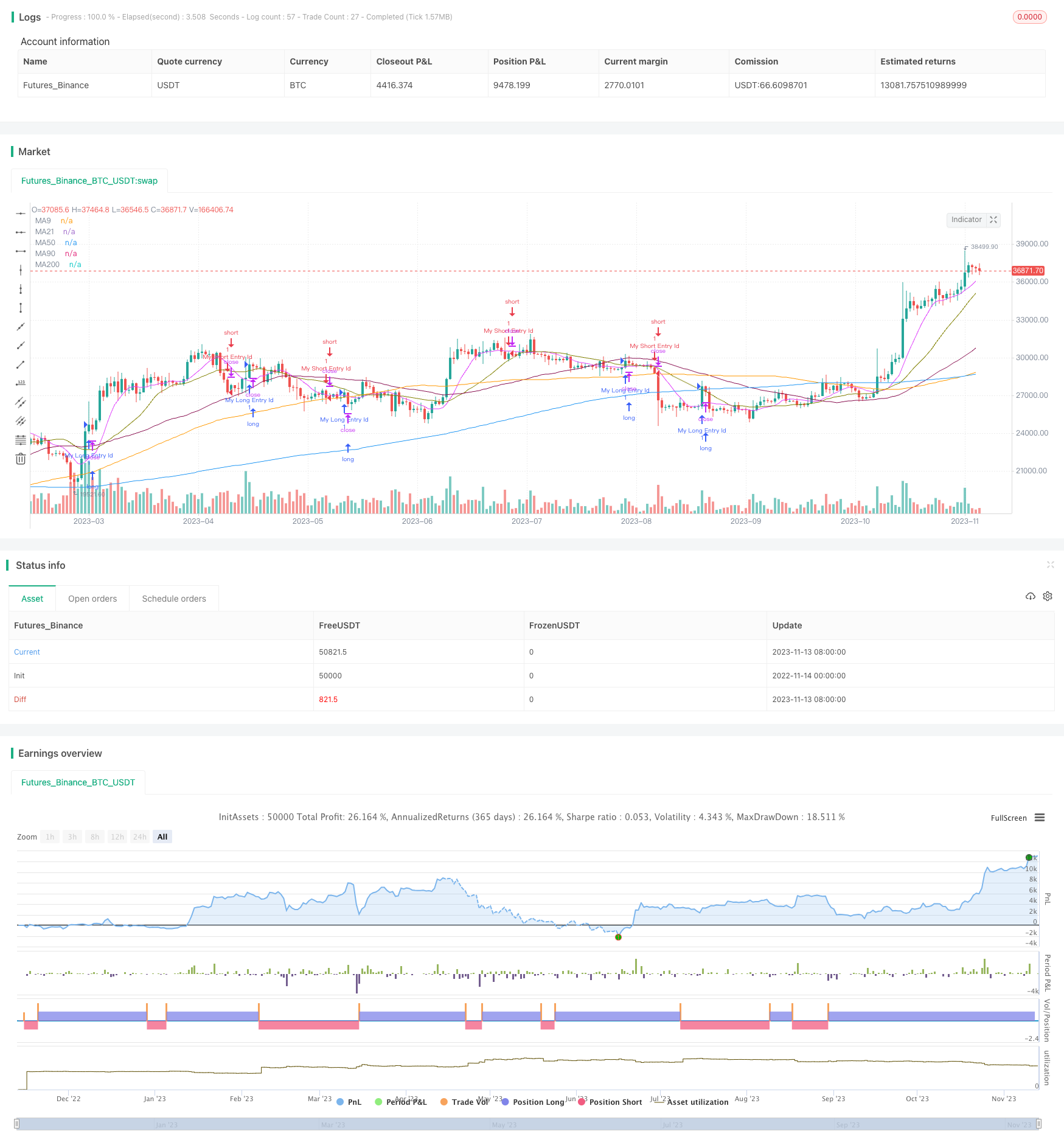# Altered OBV and MACD Quantitative Trading Strategy

Author: ChaoZhang, Date: 2023-11-15 17:58:42
Tags:## Overview

This strategy uses altered On Balance Volume (OBV) and MACD to generate trading signals. It combines price index MACD and altered OBV as a comprehensive indicator for volume and price, aiming to capture trading opportunities when price and volume strengths breakout.

## Strategy Logic

1. Calculate Simple Moving Average (SMA) to determine market trend.

2. Calculate altered OBV. It modifies the OBV calculation based on close price and previous close price relationship to make OBV more sensitive.

3. Calculate MACD on altered OBV. MACD consists of fast line, slow line and histogram to identify volume momentum change.

4. When MACD golden cross and goes up, a buy signal is generated.

5. When MACD dead cross and goes down, a sell signal is triggered.

6. Check SMAs to avoid unnecessary trades during trendless market.

1. Altered OBV is more sensitive to capture early volume changes.

2. MACD clearly indicates volume momentum change and key levels.

3. Volume and price combined signal improves accuracy.

4. SMA filters false signal by determining market trend.

5. Clear strategy logic and big optimization space.

## Risk Analysis

1. Altered OBV may generate false signals, needs filter by other indicators.

2. Improper MACD parameters setting may miss trades or cause false signals.

3. Pay attention to stock specifics to avoid losses.

4. Monitor market condition as strategy may not work for special scenarios.

5. Backtest overfit risk may lead to worse performance in live trading.

## Optimization Directions

1. Test different SMA period combinations to optimize market trend determination.

2. Test MACD parameters to better identify volume momentum change.

3. Add other indicators as filter, like KDJ, RSI etc.

5. Optimize money management to improve overall profitability.

6. Test parameter differences among stocks.

## Conclusion

The strategy combines altered OBV and MACD to achieve volume and price synthesis. It can capture volume momentum change early and generate trading signals. Compared to using OBV or MACD alone, this strategy provides more reliable trading opportunities. However, false signals risk exists and further optimizations on indicators and parameters, plus money management are needed to obtain steady profits in live trading. Overall, the strategy has clear logic and is worth testing and optimizing to explore its potential.

```/*backtest
start: 2022-11-14 00:00:00
end: 2023-11-14 00:00:00
period: 1d
basePeriod: 1h
exchanges: [{"eid":"Futures_Binance","currency":"BTC_USDT"}]
*/

// This source code is subject to the terms of the Mozilla Public License 2.0 at https://mozilla.org/MPL/2.0/

//@version=5
strategy("Altered OBV On MACD", overlay=true, margin_long=100, margin_short=100)

// This source code is subject to the terms of the Mozilla Public License 2.0 at https://mozilla.org/MPL/2.0/
//@version=5
//SMA Tredline
out = ta.sma(close, 200)
outf = ta.sma(close, 50)
outn = ta.sma(close, 90)
outt = ta.sma(close, 21)
outthree = ta.sma(close, 9)
//sma plot
offset = input.int(title="Offset", defval=0, minval=-500, maxval=500)
plot(out, color=color.blue, title="MA200", offset=offset)
plot(outf, color=color.maroon, title="MA50", offset=offset)
plot(outn, color=color.orange, title="MA90", offset=offset)
plot(outt, color=color.olive, title="MA21", offset=offset)
plot(outthree, color=color.fuchsia, title="MA9", offset=offset)

fast_length = input(title="Fast Length", defval=12)
slow_length = input(title="Slow Length", defval=26)
chng = 0
obv = ta.cum(math.sign(ta.change(close)) * volume)
if close < close and (open < close)
chng := 1
else if close > close
chng := 1
else
chng := -1
obvalt = ta.cum(math.sign(chng) * volume)
//src = input(title="Source", defval=close)
src = obvalt
signal_length = input.int(title="Signal Smoothing",  minval = 1, maxval = 50, defval = 9)
sma_source = input.string(title="Oscillator MA Type",  defval="EMA", options=["SMA", "EMA"])
sma_signal = input.string(title="Signal Line MA Type", defval="EMA", options=["SMA", "EMA"])

// Calculating
fast_ma = sma_source == "SMA" ? ta.sma(src, fast_length) : ta.ema(src, fast_length)
slow_ma = sma_source == "SMA" ? ta.sma(src, slow_length) : ta.ema(src, slow_length)
macd = fast_ma - slow_ma
signal = sma_signal == "SMA" ? ta.sma(macd, signal_length) : ta.ema(macd, signal_length)
hist = macd - signal
//hline(0, "Zero Line", color=color.new(#787B86, 50))
//plot(hist, title="Histogram", style=plot.style_columns, color=(hist>=0 ? (hist < hist ? col_grow_above : col_fall_above) : (hist < hist ? col_grow_below : col_fall_below)))
//plot(macd, title="MACD", color=col_macd)
//plot(signal, title="Signal", color=col_signal)
[macdLine, signalLine, histLine] = ta.macd(close, 12, 26, 9)
mafentry =ta.sma(close, 50) > ta.sma(close, 90)
//matentry = ta.sma(close, 21) > ta.sma(close, 50)
matwohun = close > ta.sma(close, 200)
twohunraise = ta.rising(out, 2)
twentyrise = ta.rising(outt, 2)
macdrise = ta.rising(macd,2)
macdlong = ta.crossover(macd, signal)
longCondition=false
if macdlong and macdrise
longCondition := true

if (longCondition)
strategy.entry("My Long Entry Id", strategy.long)
//Sell Signal
mafexit =ta.sma(close, 50) < ta.sma(close, 90)
matexit = ta.sma(close, 21) < ta.sma(close, 50)
matwohund = close < ta.sma(close, 200)
twohunfall = ta.falling(out, 3)
twentyfall = ta.falling(outt, 2)
shortmafall = ta.falling(outthree, 1)
macdfall = ta.falling(macd,1)
macdsell = macd < signal
shortCondition = false
if macdfall and macdsell and (macdLine < signalLine) and ta.falling(low,2)
shortCondition := true

if (shortCondition)
strategy.entry("My Short Entry Id", strategy.short)

```

More